# Setting up a Calculated Value

• 5 replies
• 48 views

• 74 replies

Hello Everyone,

I hope Monday is off to a good start for everyone.

Question: Setting up a "Calculated Value."  I'm using two "Single Line Textboxes" to multiply two numbers, to come to an "Annual Savings" Calculated Value.  I put the amount in, yet the amount does not calculate or return in the "Annual Savings" Control.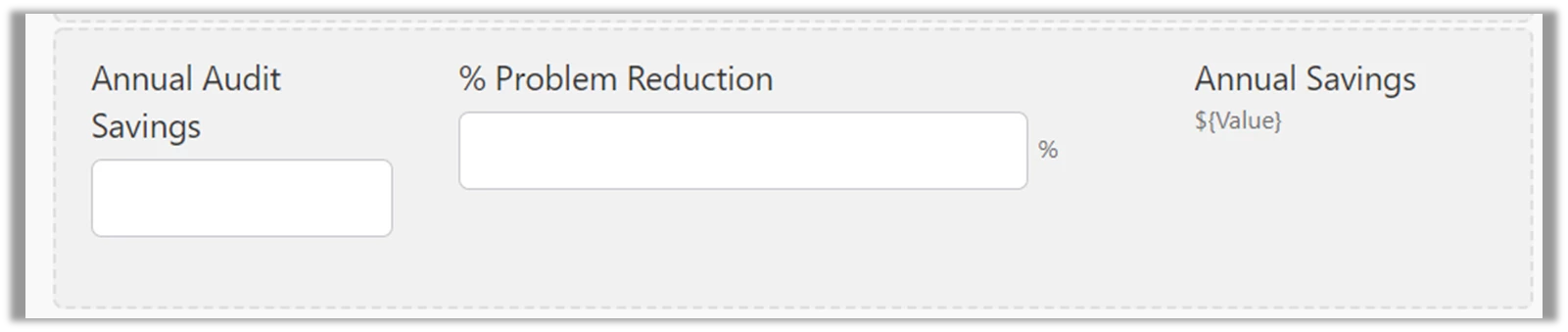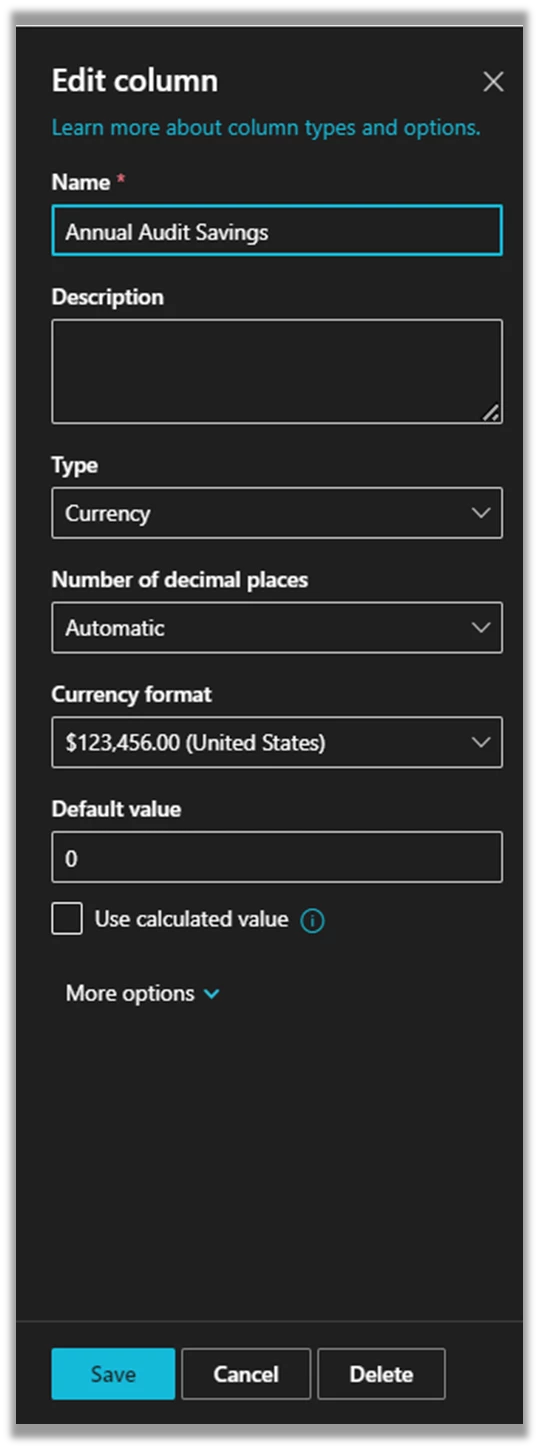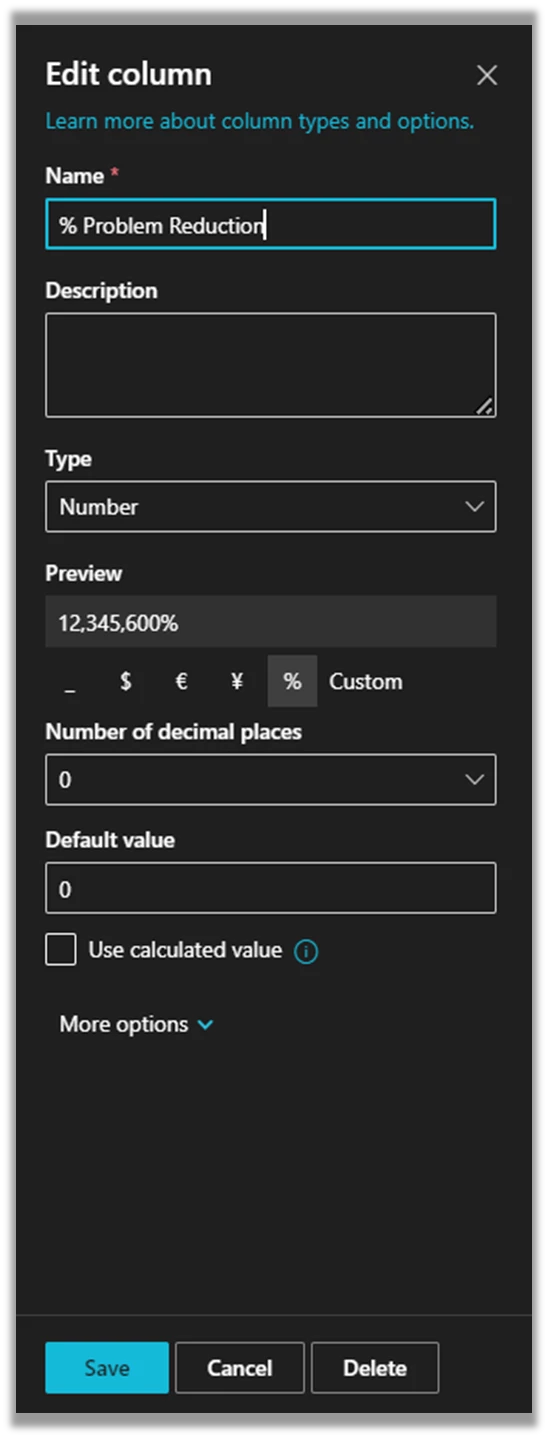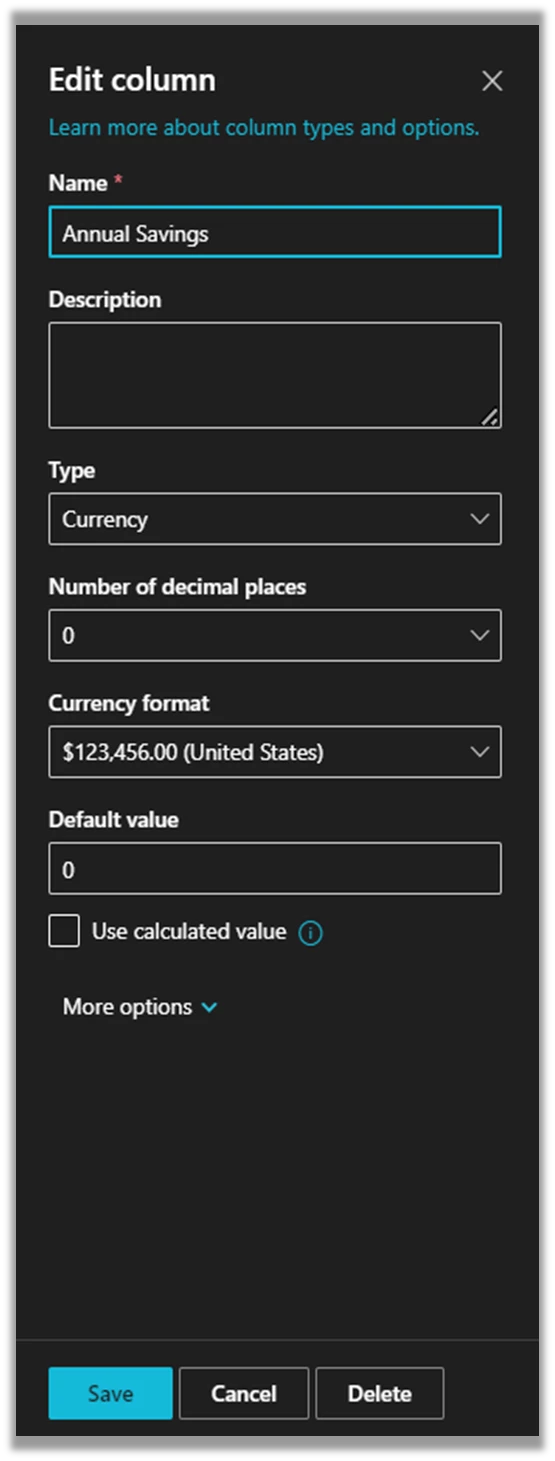Thoughts?

Thanks!

### 5 replies

Userlevel 6+14

The Annual Savings will NEVER show on the form as the values have yet to be saved into the SPO List.

What you are showing is SPO columns settings.

Are you are using SPO Calculated Value? Is this what you want?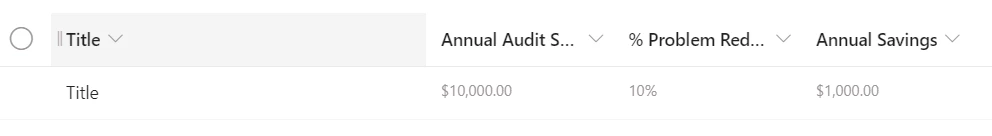The SPO calculated function will only do the "calculation" once the form is submitted (the 2 fields value saved into SPO)

Here is how to create the SPO Calculated Field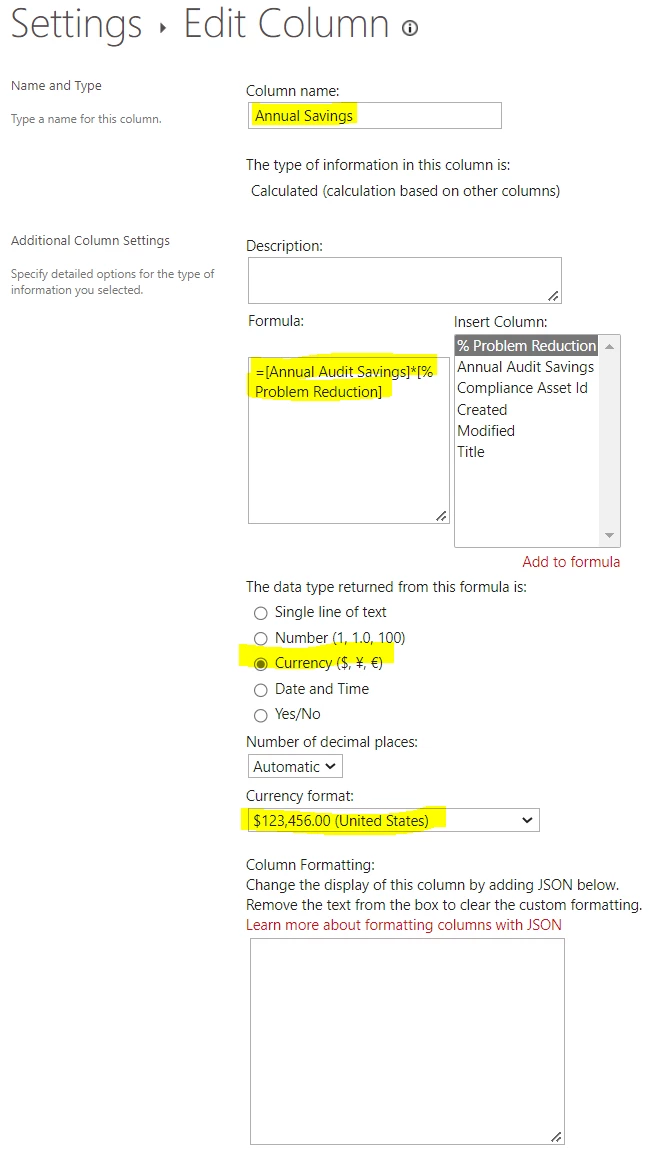Initially, I would like the calculation to show up on the Form.  For instance, in an area preceding my problem, the calculation process works and shows on the Form.  I would like that to work the same further down the Form.

This is where it works on the Form: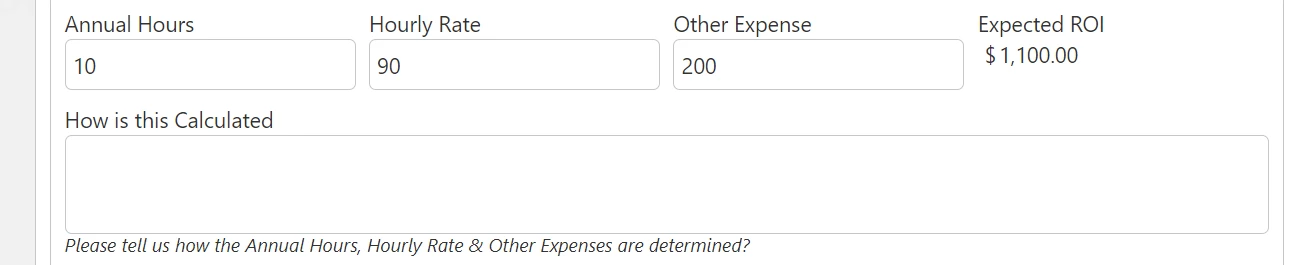This where I would like it to work on the Form (where it is not calculating, and I cannot figure out why):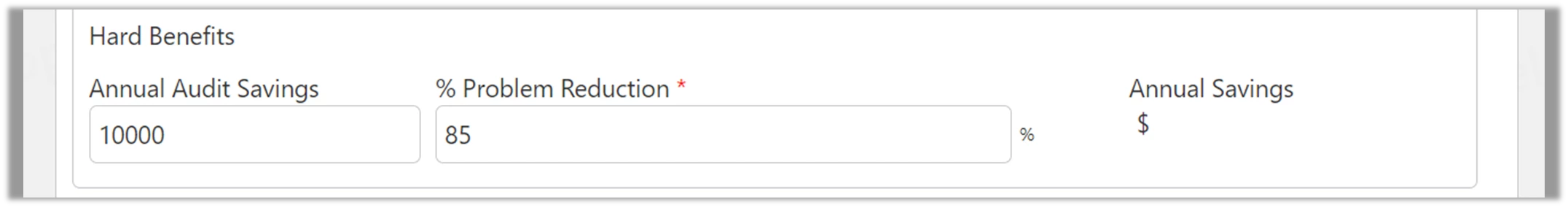Userlevel 6+14

Create a Variable to store the calculated value.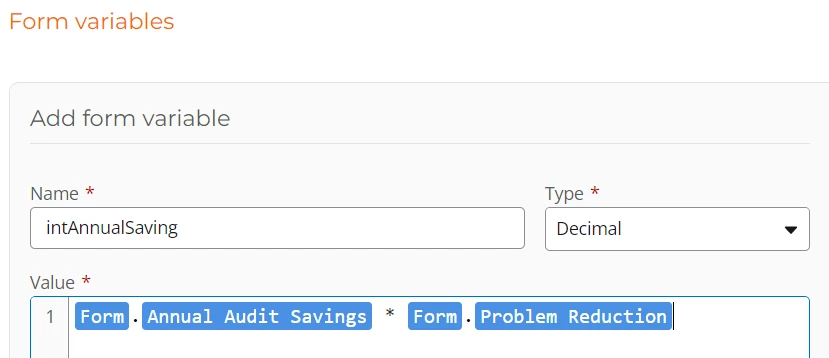Add the following to make the Problem Reduction value as a Percentage (x/100)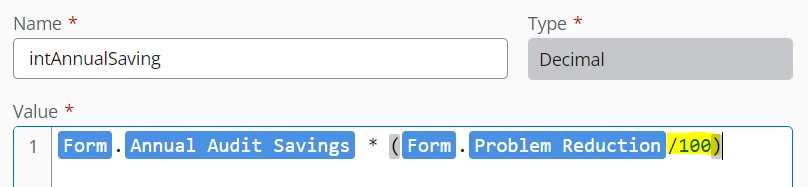Insert the recently created Variable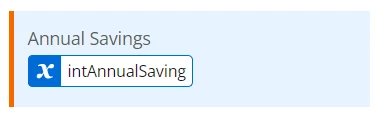Preview the form. Test with some values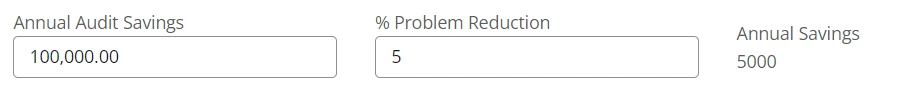Cheers

Where does the "Form" come from?  I see the "Named Controls" Annual Audit Savings & Problem Reduction.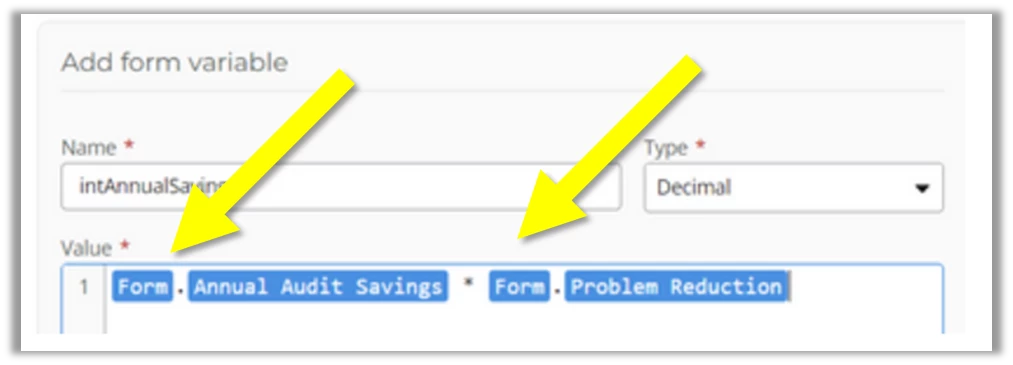Userlevel 6+14

Oops! sorry mate.

That is from the New Responsive form. Which form are you using?

Responsive or Classic?

Use the Named controls.

I'm using a Calculated Value control to  display the calculations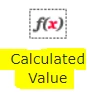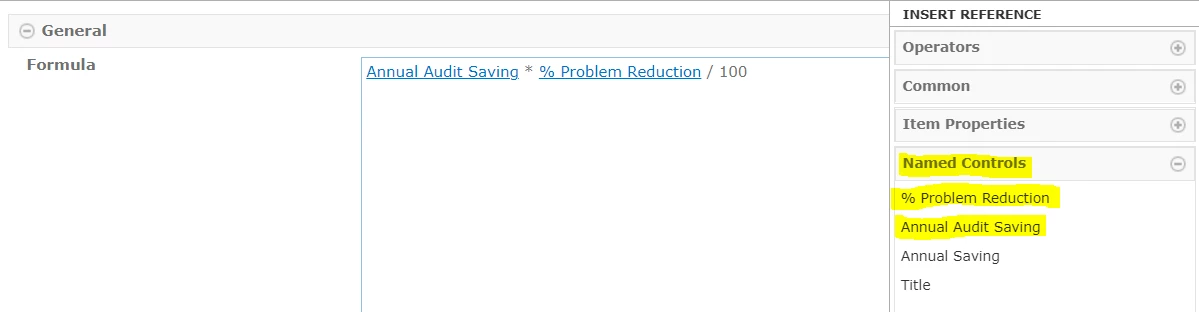Hope this helps